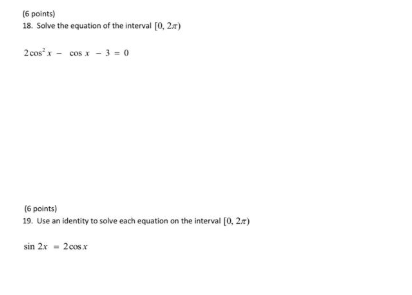# 9(tan(2a)+2cos(a)=0 interval 0 less than or equal to a less than or equal to 2pi

9(tan(2a)+2cos(a)=0 interval 0 less than or equal to a less than or equal to 2pi

Solve each equation...trig equations for linear sin and cos

This Homework Help Question: "9(tan(2a)+2cos(a)=0 interval 0 less than or equal to a less than or equal to 2pi" No answers yet.

We need 10 more requests to produce the answer to this homework help question. Share with your friends to get the answer faster!

0 /10 have requested the answer to this homework help question.

Once 10 people have made a request, the answer to this question will be available in 1-2 days.
All students who have requested the answer will be notified once they are available.
##### Add Answer of: 9(tan(2a)+2cos(a)=0 interval 0 less than or equal to a less than or equal to 2pi
Similar Homework Help Questions
• ### Solve: sin x - 2sin x cos x = 0 for 0 is less than or equal to x is less than 2pi

Solve: sin x - 2sin x cos x = 0 for 0 is less than or equal to x is less than 2pi.

• ### Solve the following equation for 0 less than and/or equal to "x" less than and/or equal to 360 -- cos^2x - 1 = sin^2x -- Attempt: cos^2x - 1 - sin^2x = 0 cos^2x - 1 - (1 - cos^2x) = 0 cos^2x - 1 - 1 + cos^2x = 0 2cos^2x - 2 = 0 (2cos^2x/2)= (-2/2) cos^

Solve the following equation for 0 less than and/or equal to "x" less than and/or equal to 360--cos^2x - 1 = sin^2x--Attempt:cos^2x - 1 - sin^2x = 0cos^2x - 1 - (1 - cos^2x) = 0cos^2x - 1 - 1 + cos^2x = 02cos^2x - 2 = 0(2cos^2x/2)= (-2/2)cos^2x = -1cosx = square root of -1And I can't do anything with this now...what am I doing wrong?--Textbook answers:0, 180, 360--

• ### Solve: 2 cos2 x - 3 cos x + 1 = 0 for 0 is less than or equal to x is less than 2pi

Solve: 2 cos2 x - 3 cos x + 1 = 0 for 0 is less than or equal to x is less than 2pi.

• ### trig question 2sin^2x+sinx-6=0 how to solve for x when the restriction is x is greater than or equal to zero but less than 2pi

trig question2sin^2x+sinx-6=0how to solve for x when the restriction is x is greater than or equal to zero but less than 2pi

• ### Solve for x in the equation 3sin^2x = cos^2x; 0<or=x<or =2pi 3 sin squared x = cos squared x where x is greater than or = 0 and less than or = 2pi Thank you so much for your help

Solve for x in the equation 3sin^2x = cos^2x; 0

• ### 16 points) 18. Solve the equation of the interval [0, 2x) 2cos - Cos - 3...16 points) 18. Solve the equation of the interval [0, 2x) 2cos - Cos - 3 = 0 (6 points) 19. Use an identity to solve each equation on the interval [0, 2.1) sin 2x - 2006

• ### 16 points) 18. Solve the equation of the interval [0, 2x) 2cos - Cos - 3...16 points) 18. Solve the equation of the interval [0, 2x) 2cos - Cos - 3 = 0 (6 points) 19. Use an identity to solve each equation on the interval [0, 2.1) sin 2x - 2006

• ### Solve each equation for zero less than equal to x less than 2pi

tan(x)^2=2tanxsinxsin(x)^2+sin(x)-1=03coscotx+7=5csc(x)2cos(x)^2-cos(x)=2-sec(x)I've tried to solve these problems so many time but can seem to figure out how to do it. I havethe ansers. i guess its just the alebraic manipulation the isthe problem .

• ### If 2cos A-1=0 where 0<or = A<2pi then what is A equal to

If 2cos A-1=0 where 0<or = A<2pi then what is A equal to?

• ### Solve the equation for x in the interval 0<x<2pi 1/ 1+tan^2x = -cos x How would i do this

Solve the equation for x in the interval 0<x<2pi1/ 1+tan^2x = -cos x How would i do this? im thinking of maybe changing the 1+tan to sec^2x?

Free Homework App